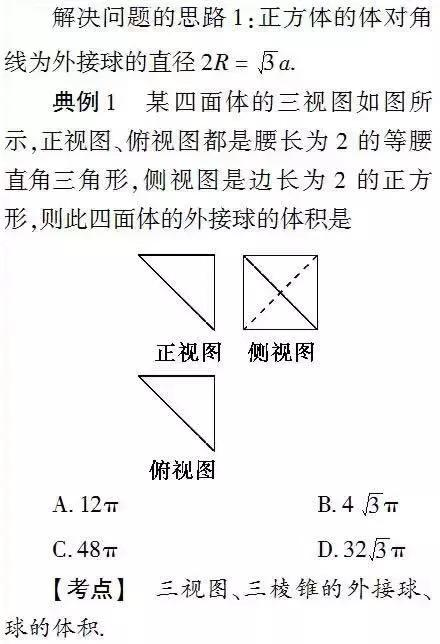# 怎么样计算球体的半径 应该怎么做？wikiHow的内容管理团队会严密监督编辑人员的工作，确保每篇文章都符合我们的高质量标准要求。#include<stdio.h>　　float GV(float x)　　{### 方法 1使用半径计算公式1知道直径的情况下求半径。半径是直径的一半，所以请使用公式r = D/2。这与根据圆形直径计算其半径的方法相同。2知道周长的情况下求半径。请使用公式C/2π。由于周长等于πD，等于2πr，所以用周长除以2π后即可求得半径。3知道球体体积的情况下计算半径。使用公式((V/π)(3/4))1/3球体的体积是根据公式V = (4/3)πr3计算得出的。在这个公式中解变量r可得((V/π)(3/4))1/3 = r，这意味着球体的半径等于体积除以π，乘以3/4，再整体求1/3次幂或立方根。

((V/π)(3/4))1/3 = r

((100/π)(3/4))1/3 = r

((31.83)(3/4))1/3 = r

(23.87)1/3 = r

2.88 cm = r4根据表面积求半径。请使用公式r = √(A/(4π))。球体的表面积是根据公式A = 4πr2进行计算的。解变量r得到√(A/(4π)) = r，这意味着球体的半径等于表面积除以4π后的平方根。你还可以取(A/(4π))的1/2次幂，来求得相同的结果。

√(A/(4π)) = r

√(1200/(4π)) = r

√(300/(π)) = r

√(95.49) = r

9.77 cm = r

### 方法 2定义关键概念1确定球体的基本尺寸。半径r是球体准确中心到其表面任意一点的距离。一般来说，如果知道球体的直径、周长、体积或表面积，你就能求出它的半径。

π：表示圆形周长和圆形直径之比的常数。π的前十位数等于3.141592653，通常四舍五入成3.142使用各种尺寸来计算半径。你可以使用直径、周长、体积和表面积来计算球体的半径。如果知道半径本身的长度，你还可以根据它来计算上述各项数值。因此，为了求得半径，请试着变换计算这些数值的公式。学习那些使用半径计算直径、周长、体积和表面积的公式。

D = 2r。和圆形一样，球体的直径是半径的两倍。

C = πD或2πr。和圆形一样，球体的周长等于π乘以直径。由于直径是半径的两倍，所以我们也可以说周长等于两倍的半径乘以π。

V = (4/3)πr3。球体的体积等于半径的立方乘以π，再乘以4/3。立方指的是一个数字乘以它自身两次。

A = 4πr2。球体的表面积等于半径的平方乘以π，再乘以4。平方指的是一个数字乘以它自身。由于圆形的面积等于πr2，所以我们也可以说球体的表面积是其周长形成的圆的面积的四倍。

### 方法 3计算作为两点之间距离的半径1求球体中心点的(x,y,z)坐标。我们可以将球体的半径看作是球体中心点到球体表面任意点的距离。因为以上陈述为真，所以如果知道球体中心点和表面任意点的坐标，那么使用变形后的基本距离公式就能计算出两点之间的距离，从而求得球体的半径。首先，求得球体中心点的坐标。注意，由于球体是三维图形，其中心点的坐标会是(x,y,z)，而不是(x,y)。2求得球体表面一点的坐标。然后你需要求得球体表面一点的(x,y,z)坐标。这个点可以是球体表面的任意一点。由于根据定义，球体表面上所有点到中心点的距离都是相等的，所以任意一点都可以用来确定半径。3使用公式d = √((x2 - x1)2 + (y2 - y1)2 + (z2 - z1)2)来求得半径。知道球体中心点和表面点的坐标后，计算两点之间的距离可以求出半径。使用三维距离公式d = √((x2 - x1)2 + (y2 - y1)2 + (z2 - z1)2)来计算两点之间的距离，其中d等于距离，(x1,y1,z1)等于中心点的坐标，而(x2,y2,z2)等于表面点的坐标。

d = √((x2 - x1)2 + (y2 - y1)2 + (z2 - z1)2)

d = √((3 - 4)2 + (3 - -1)2 + (0 - 12)2)

d = √((-1)2 + (4)2 + (-12)2)

d = √(1 + 16 + 144)

d = √(161)

d = 12.69。这个值就是本题球体的半径。4要知道，一般情况下r = √((x2 - x1)2 + (y2 - y1)2 + (z2 - z1)2)。在球体中，表面每一点到中心点的距离都是相等的。取上述三维距离公式，并用半径r变量代替d变量后，可以得到一个变形公式，已知任意中心点(x1,y1,z1)和任意对应表面点(x2,y2,z2)时，我们可以使用这个公式来计算半径。

## 小提示

π是希腊字母，代表圆形周长和其直径的比值。它是一个无理数，不能写成两个整数之比，但它存在许多近似值，333/106可以给出π小数点后的四位数。如今，大多数人都会记住π的近似值3.14，对于日常使用来说，这个值通常足够精确。

## 参考

↑ http://www.rkm.com.au/CALCULATORS/CALCULATOR-circle-sphere.html↑ http://www.calculatorsoup.com/calculators/geometry-solids/sphere.php↑ http://www.varsitytutors.com/sat_math-help/how-to-find-the-radius-of-a-sphere↑ http://mathcentral.uregina.ca/QQ/database/QQ.09.07/h/cey2.html↑ http://formulas.tutorvista.com/math/sphere-formula.html↑ http://www.web-formulas.com/Math_Formulas/Geometry_Volume_of_Sphere.aspx↑ http://mathforum.org/library/drmath/view/54892.html

## 相关wikiHowsV=（1/6）πd^3 (六分之一乘以π乘以直径的三次方)

#include<stdio.h>

int main(void)

{

int r;

double v;//这个变量应该改为double类型

r=10;

v=4.0f/3.0f*3.14*r*r*r;

printf("V: %lf\n",v);//这里的输出格式应该为%lf,不是%d

return 1;//这里有一个返回值，因为你的主函数定义的有int类型的返回值

}